Courses

# Graphs Algorithms MCQ - 2

## 25 Questions MCQ Test GATE Computer Science Engineering(CSE) 2022 Mock Test Series | Graphs Algorithms MCQ - 2

Description
This mock test of Graphs Algorithms MCQ - 2 for Computer Science Engineering (CSE) helps you for every Computer Science Engineering (CSE) entrance exam. This contains 25 Multiple Choice Questions for Computer Science Engineering (CSE) Graphs Algorithms MCQ - 2 (mcq) to study with solutions a complete question bank. The solved questions answers in this Graphs Algorithms MCQ - 2 quiz give you a good mix of easy questions and tough questions. Computer Science Engineering (CSE) students definitely take this Graphs Algorithms MCQ - 2 exercise for a better result in the exam. You can find other Graphs Algorithms MCQ - 2 extra questions, long questions & short questions for Computer Science Engineering (CSE) on EduRev as well by searching above.
*Answer can only contain numeric values
QUESTION: 1

### Consider the following directed graph: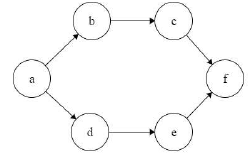The number of different topological orderings of the vertices of the graph is _____________.

Solution:

Here Start with a and End with f.

a _ _ _ _ f

Blanks spaces are to be filled with b, c, d, e such that b comes before c, and d comes before e..
Number of ways to arrange b,c,d,e such that b comes before c and d comes before e. will be = 4!/(2!*2!) = 6

*Answer can only contain numeric values
QUESTION: 2

### Breadth First Search (BFS) is started on a binary tree beginning from the root vertex. There is a vertex t  at a distance four from the root. If t is the  n-th vertex in this BFS traversal, then the maximum possible value of n is _________.

Solution:

No of nodes at level 0(root) of tree =>1
No of nodes at level 1 of tree =>2
No of nodes at level 2 of tree =>4
No of nodes at level 3 of tree =>8
No of nodes at level 4 of tree =>16
Last node in level 4th is the node we are looking for => 1+2+4+8+16 => 31

QUESTION: 3

### Which of the following statements is false?

Solution:

(a) True.
(b) False.
(c) True.
(d) True.

QUESTION: 4

Which one of the following algorithm design techniques is used in finding all pairs of shortest distances in a graph?

Solution:

Answer is A because floyd warshall algorithm used to find all shortest path which is a dynamic programming approach.

QUESTION: 5

The most appropriate matching for the following pairs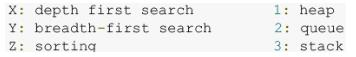is:

Solution:

X - 3 DFS uses stack implicitly

Y-2 BFS uses queue explicitly in Algo
Z-1 Heap-Heapsort

QUESTION: 6

Consider an undirected unweighted graph G. Let a breadth-first traversal of G be done starting from a node r. Let d(r,u) and d(r,v) be the lengths of the shortest paths from r to u and v respectively in G. if u visited before v during the breadth-first traversal, which of the following statements is correct?

Solution:

BFS is used to count shortest path from source (If all path costs are 1 !)
now if u is visited before v it means 2 things.
1. Either u is closer to v.
2. if u & v are same distance from r, then our BFS algo chose to visit u before v.

QUESTION: 7

Consider the following graph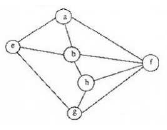Among the following sequences

I. abeghf
II. abfehg
III. abfhge
IV. afghbe

which are depth first traversals of the above graph?

Solution:

DFS goes upto how much depth possible and then backtrack and go to the next link.

Here only 'abfehg' not possible because e and h consecutively is not possible by any backtracking of DFS traversal

QUESTION: 8

Suppose we run Dijkstra’s single source shortest-path algorithm on the following edge-weighted directed graph with vertex P as the source.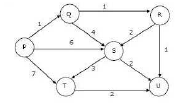In what order do the nodes get included into the set of vertices for which the shortest path distances are finalized?

Solution:

In Dijkstra's algorithm at each point we choose the smallest weight edge which starts from any one of the vertices in the shortest path found so far and add it to the shortest path.

QUESTION: 9

Let G1 = (V, E1) and G2 = (V, E2)  be connected graphs on the same vertex set V with more than two vertices. If   G1 ∩ G2  = (V, E∩ E2 )  is not a connected graph, then the graph G1 ∪ G2  = (V, E∪ E2 )

Solution:

Take a tree for example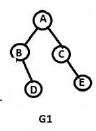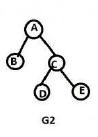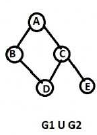(A) False. Every vertex of tree(other than leaves) is a cut vertex
(B)True
(C)False. Without E in G1 and G2, G1 U G2 has no bridge.
(D)False. G1 U G2, G1, G2 three graphs have same chromatic number of 2.

QUESTION: 10

Consider the undirected graph below: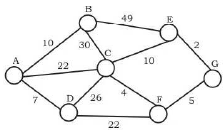Using Prim's algorithm to construct a minimum spanning tree starting with node A, which one of the following sequences of edges represents a possible order in which the edges would be added to construct the minimum spanning tree?

Solution:

(A) and (B) produce disconnected components with the GIVEN order in options which is NEVER allowed by
prims's algorithm.
(C) produces connected component every instant a new edge is added BUT when first vertex is chosen(first vertex is chosen randomly) first edge must be the minimum weight edge that is chosen . Therefore (A,D)
MUST be chosen BEFORE (A,B). Therefore (C) is FALSE

QUESTION: 11

Let S and t be two vertices in a undirected graph G=(V,E) having distinct positive edge weights. Let [X,Y] be a partition of  V such that  s ∈ X and t ∈ Y . Consider the edge  having the minimum  weight amongst all those edges that have one vertex in X and one vertex in Y

The edge e must definitely belong to:.

Solution:

For 82a The answer should be Option A because edge 'e' is the lightest safe edge connecting X and Y so the minimum
spanning tree of G must contain 'e' (Greedy and optimal choice).
While B might seem correct but it is not always true. One such case is when G is not connected therefore there might not
be any path between 's' and 't'.
Since the question is about definitely true B is incorrect and A is the only correct option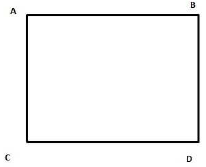Lets say AC =1 CD = 2 BD = 3 and AB=4

Then if s= A and t= B then AC is the lightest edge crossing X and Y where X = { A } and Y = { C, B, D}
But clearly AC is not on the shortest path from A to B. The shortest path is AB = 4.

QUESTION: 12

In a depth-first traversal of a graph G with n vertices, K edges are marked as tree edges. The number of connected components in G is

Solution:

Tree edges are the edges that are part of DFS tree.  If there are x tree edges in a tree, then  x+1 vertices in the tree.

The output of DFS is a forest if the graph is disconnected.  Let us see below simple example where graph is disconnected.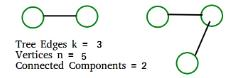QUESTION: 13

In the following table, the left column contains the names of standard graph algorithms and the right column contains the time complexities of the algorithms. Match each algorithm with its time complexity.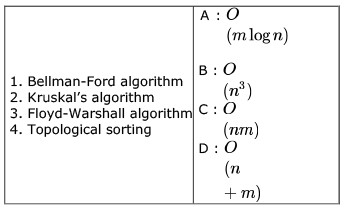Solution:

1. Bellman-Ford algorithm =>: O (nm) Assuming n as edges , m as vertices, for every vertex we relax all edges. m*n ,
O(mn)
2. Kruskal’s algorithm => Remaining Option ,A : O ( m log n)
3. Floyd-Warshall algorithm => Dynamic Programming Algo, O(N3)
4. Topological sorting => Boils Down to DFS, O(V+E) D

QUESTION: 14

To implement Dijkstra’s shortest path algorithm on unweighted graphs so that it runs in linear time, the data structure to be used is:

Solution:

we can find single source shortest path in unweighted graph by using Breadth first search (BFS) algorithm which using "Queue" data structure , which time O(m+n) (i.e. linear with respect to the number of vertices and edges. )

QUESTION: 15

Let T be a depth first search tree in an undirected graph G. Vertices u and ν are leaves of this tree T. The degrees of both u and ν in G are at least 2. which one of the following statements is true?

Solution: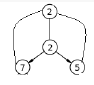Consider following graph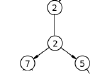Dfs is .
so D is answer.

QUESTION: 16

Which of the following is the correct decomposition of the directed graph given below into its strongly connected components?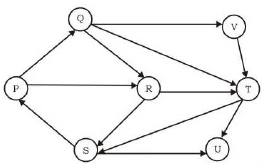Solution:

A graph is said to be strongly connected if every vertex is reachable from every other vertex.

The strongly connected component is always maximal that is if x is strongly connected component there should not exist another strongly connected component which contains x.

If we take R as a strongly connected component but which is part of PQRS and PQRS is part of PQRSVT.

QUESTION: 17

Consider the depth-first-search of an undirected graph with 3 vertices P, Q, and R. Let discovery time d(u) represent the time instant when the vertex u is first visited, and finish time f(u) represent the time instant when the vertex u is last visited. Given that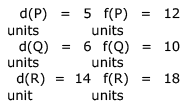Which one of the following statements is TRUE about the graph

Solution: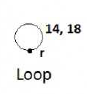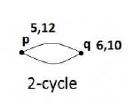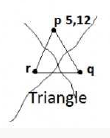As seen in quetion after 10 we have to go for p again and since p is finish and then r is start so r must be disconnected because if their is edges from q to r then r must be visited before of q and p ending.

QUESTION: 18

In an unweighted, undirected connected graph, the shortest path from a node S to every other node is computed most efficiently, in terms of time complexity, by

Solution:

Dijkastra and warshall 's algo only used for weighted graph.

Both DFS and BFS can be used for finding path between 2 vertices in undirected and unweighted graph but BFS can only give the shortest path as concern in given question so BFS is Ans.

Note :- finding only path(DFS) and finding shortest path(BFS) ..Matters a lot

QUESTION: 19

Consider the DAG with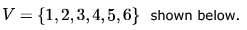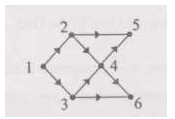Which of the following is not a topological ordering?

Solution:

Go with Vertex with indegree 0 remove that vertex with all edges foing from it . Follow that procedure.
We See 3 cannot come at first B/c indegree is not 0. so D is answer here .
ALL other options are Topologocal order.
Only 1 and 4 order matter for this quetion.

QUESTION: 20

A depth-first search is performed on a directed acyclic graph. Let d[u] denote the time at which vertex u is visited for the first time and f[u] the time at which the dfs call to the vertex u terminates. Which of the following statements is always true for all edges (u, v) in the graph ?

Solution: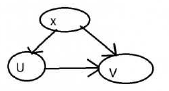I'm gonna disprove all wrong options here.
A) d[u] < d[v] , Counter Example => Well if we directly start DFS on V first. Then I call DFS on X which visits U.
B) d[u] < f[v] Counter example => Same as A)
C) f[u] < f[v] Counter example => Same as A) again
So answer is D)

QUESTION: 21

Consider a weighted undirected graph with positive edge weights and let  be an edge in the graph. It is known that the shortest path from the source vertex  to  has weight 53 and the shortest path from  to  has weight 65. Which one of the following statements is always true?

Solution: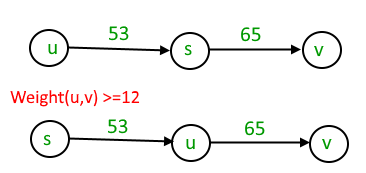The minimum weight happens when (S,U) + (U,V) = (S,V)

Else (S,U) + (U,V) >= (S,V)

Given (S,U) = 53, (S,V) = 65

53 + (U,V) >= 63

(U,V) >= 12.

QUESTION: 22

The Breadth First Search algorithm has been implemented using the queue data structure. One possible order of visiting the nodes of the following graph is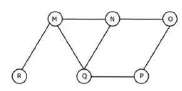Solution:

A) MNOPQR -> If you try to run BFS, after M, you must traverse NQR (In some order) Here P is traversed before Q, which
is wrong.
B) NQMPOR -> This is also not BFS. P is traversed before O !
C) QMNPRO -> Correct
D) QMNPOR -> Incorrect. Because R need to be traversed before O.(Because M is ahead of N in queue.
Answer :- > C

QUESTION: 23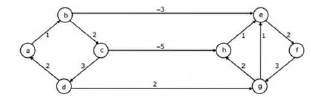Dijkstra's single source shortest path algorithm when run from vertex  in the above graph, computes the correct shortest path distance to

Solution:

(d) all the vertices. Just simulate the Dijkstra's algorithm on it. Dijkstra's algorithm is not meant for graphs with negative edge- weight-cycle, but here it does give the correct shortest path.

QUESTION: 24

The most efficient algorithm for finding the number of connected components in an undirected graph on  vertices and edges has time complexity

Solution:

Run DFS to find connected components. Its time complexity is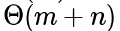QUESTION: 25

Consider the following sequence of nodes for the undirected graph given below.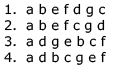A Depth First Search (DFS) is started at node a. The nodes are listed in the order they are first visited. Which all of the above is (are) possible output(s)?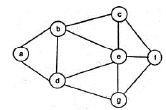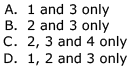Solution:

1. After f is visited, c or g should be visited next. So, the traversal is incorrect.
4. After c is visited, e or f should be visited next. So, the traversal is incorrect.
2 and 3 are correct.